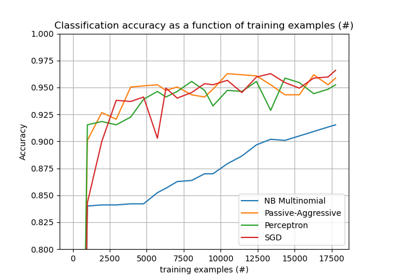# sklearn.naive_bayes.MultinomialNB¶

class sklearn.naive_bayes.MultinomialNB(*, alpha=1.0, fit_prior=True, class_prior=None)[source]

Naive Bayes classifier for multinomial models.

The multinomial Naive Bayes classifier is suitable for classification with discrete features (e.g., word counts for text classification). The multinomial distribution normally requires integer feature counts. However, in practice, fractional counts such as tf-idf may also work.

Read more in the User Guide.

Parameters:
alphafloat, default=1.0

Additive (Laplace/Lidstone) smoothing parameter (0 for no smoothing).

fit_priorbool, default=True

Whether to learn class prior probabilities or not. If false, a uniform prior will be used.

class_priorarray-like of shape (n_classes,), default=None

Prior probabilities of the classes. If specified, the priors are not adjusted according to the data.

Attributes:
class_count_ndarray of shape (n_classes,)

Number of samples encountered for each class during fitting. This value is weighted by the sample weight when provided.

class_log_prior_ndarray of shape (n_classes,)

Smoothed empirical log probability for each class.

classes_ndarray of shape (n_classes,)

Class labels known to the classifier

feature_count_ndarray of shape (n_classes, n_features)

Number of samples encountered for each (class, feature) during fitting. This value is weighted by the sample weight when provided.

feature_log_prob_ndarray of shape (n_classes, n_features)

Empirical log probability of features given a class, P(x_i|y).

n_features_int

DEPRECATED: Attribute n_features_ was deprecated in version 1.0 and will be removed in 1.2.

n_features_in_int

Number of features seen during fit.

New in version 0.24.

feature_names_in_ndarray of shape (n_features_in_,)

Names of features seen during fit. Defined only when X has feature names that are all strings.

New in version 1.0.

BernoulliNB

Naive Bayes classifier for multivariate Bernoulli models.

CategoricalNB

Naive Bayes classifier for categorical features.

ComplementNB

Complement Naive Bayes classifier.

GaussianNB

Gaussian Naive Bayes.

References

C.D. Manning, P. Raghavan and H. Schuetze (2008). Introduction to Information Retrieval. Cambridge University Press, pp. 234-265. https://nlp.stanford.edu/IR-book/html/htmledition/naive-bayes-text-classification-1.html

Examples

>>> import numpy as np
>>> rng = np.random.RandomState(1)
>>> X = rng.randint(5, size=(6, 100))
>>> y = np.array([1, 2, 3, 4, 5, 6])
>>> from sklearn.naive_bayes import MultinomialNB
>>> clf = MultinomialNB()
>>> clf.fit(X, y)
MultinomialNB()
>>> print(clf.predict(X[2:3]))



Methods

 fit(X, y[, sample_weight]) Fit Naive Bayes classifier according to X, y. get_params([deep]) Get parameters for this estimator. partial_fit(X, y[, classes, sample_weight]) Incremental fit on a batch of samples. Perform classification on an array of test vectors X. Return log-probability estimates for the test vector X. Return probability estimates for the test vector X. score(X, y[, sample_weight]) Return the mean accuracy on the given test data and labels. set_params(**params) Set the parameters of this estimator.
fit(X, y, sample_weight=None)[source]

Fit Naive Bayes classifier according to X, y.

Parameters:
X{array-like, sparse matrix} of shape (n_samples, n_features)

Training vectors, where n_samples is the number of samples and n_features is the number of features.

yarray-like of shape (n_samples,)

Target values.

sample_weightarray-like of shape (n_samples,), default=None

Weights applied to individual samples (1. for unweighted).

Returns:
selfobject

Returns the instance itself.

get_params(deep=True)[source]

Get parameters for this estimator.

Parameters:
deepbool, default=True

If True, will return the parameters for this estimator and contained subobjects that are estimators.

Returns:
paramsdict

Parameter names mapped to their values.

property n_features_

DEPRECATED: Attribute n_features_ was deprecated in version 1.0 and will be removed in 1.2. Use n_features_in_ instead.

partial_fit(X, y, classes=None, sample_weight=None)[source]

Incremental fit on a batch of samples.

This method is expected to be called several times consecutively on different chunks of a dataset so as to implement out-of-core or online learning.

This is especially useful when the whole dataset is too big to fit in memory at once.

This method has some performance overhead hence it is better to call partial_fit on chunks of data that are as large as possible (as long as fitting in the memory budget) to hide the overhead.

Parameters:
X{array-like, sparse matrix} of shape (n_samples, n_features)

Training vectors, where n_samples is the number of samples and n_features is the number of features.

yarray-like of shape (n_samples,)

Target values.

classesarray-like of shape (n_classes,), default=None

List of all the classes that can possibly appear in the y vector.

Must be provided at the first call to partial_fit, can be omitted in subsequent calls.

sample_weightarray-like of shape (n_samples,), default=None

Weights applied to individual samples (1. for unweighted).

Returns:
selfobject

Returns the instance itself.

predict(X)[source]

Perform classification on an array of test vectors X.

Parameters:
Xarray-like of shape (n_samples, n_features)

The input samples.

Returns:
Cndarray of shape (n_samples,)

Predicted target values for X.

predict_log_proba(X)[source]

Return log-probability estimates for the test vector X.

Parameters:
Xarray-like of shape (n_samples, n_features)

The input samples.

Returns:
Carray-like of shape (n_samples, n_classes)

Returns the log-probability of the samples for each class in the model. The columns correspond to the classes in sorted order, as they appear in the attribute classes_.

predict_proba(X)[source]

Return probability estimates for the test vector X.

Parameters:
Xarray-like of shape (n_samples, n_features)

The input samples.

Returns:
Carray-like of shape (n_samples, n_classes)

Returns the probability of the samples for each class in the model. The columns correspond to the classes in sorted order, as they appear in the attribute classes_.

score(X, y, sample_weight=None)[source]

Return the mean accuracy on the given test data and labels.

In multi-label classification, this is the subset accuracy which is a harsh metric since you require for each sample that each label set be correctly predicted.

Parameters:
Xarray-like of shape (n_samples, n_features)

Test samples.

yarray-like of shape (n_samples,) or (n_samples, n_outputs)

True labels for X.

sample_weightarray-like of shape (n_samples,), default=None

Sample weights.

Returns:
scorefloat

Mean accuracy of self.predict(X) wrt. y.

set_params(**params)[source]

Set the parameters of this estimator.

The method works on simple estimators as well as on nested objects (such as Pipeline). The latter have parameters of the form <component>__<parameter> so that it’s possible to update each component of a nested object.

Parameters:
**paramsdict

Estimator parameters.

Returns:
selfestimator instance

Estimator instance.

## Examples using sklearn.naive_bayes.MultinomialNB¶Out-of-core classification of text documents

Out-of-core classification of text documents